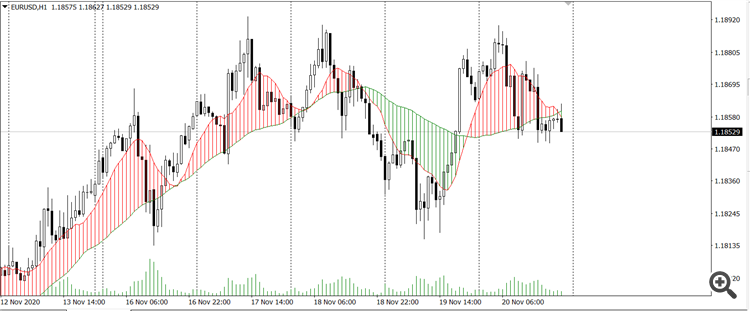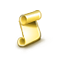Interesting script?
So post a link to it -
let others appraise it
You liked the script? Try it in the MetaTrader 5 terminal# Doulble MA Cross Draw Histogram - indicator for MetaTrader 4

Views:
4336
Rating:
Published:
2020.11.21 15:30

Use the intersection of two moving average lines to draw a vertical line.Then You can simply read the current market patternSwing trading 3 EMA Crossover

Hassane ZibaraVisual Trend Indicator

Visual multi-timeframe trend indicator.Fibonacci Potential Entry - MT4

The 8 effective steps to build a robust day trading plan using Fibonacci retracementStoch RSI MT4

The Stochastic RSI indicator (Stoch RSI) is essentially an indicator of an indicator. It is used in technical analysis to provide a stochastic calculation to the RSI indicator. This means that it is a measure of RSI relative to its own high/low range over a user defined period of time. The Stochastic RSI is an oscillator that calculates a value between 0 and 1 which is then plotted as a line. This indicator is primarily used for identifying overbought and oversold conditions.